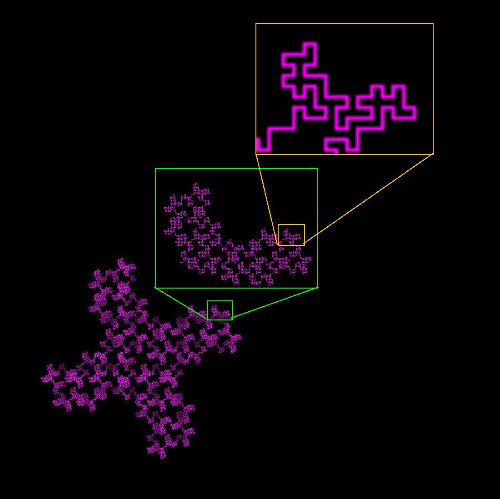רוצים לקבל ייעוץ חינם והצעת מחיר לפרויקט?
חזרה לרשימת הפרויקטים

# Calculation of images fractal properties

Image processing solution for computing fractal properties of the objects displayed on the images.

Our experts have developed a Matlab based image processing solution supporting various formats - .png, .jpg, .gif, .tiff, .bmp. The goal of the system is to compute fractal properties of objects displayed on images.

We have implemented algorithms for the following fractal dimensions: capacity dimension, correlation dimension, information probability dimensions and lacunarity and succolarity parameters.

Processing code for the first 4 parameters was converted from an existing C++ application called FDim.

Lacunarity and succolarity for 2D and 3D case were calculated by several versions of the algorithms that are described in “Using Fractal Characteristics such as Fractal Dimension, Lacunarity and Succolarity to Characterize Texture Patterns on Images” paper by R. H. Correia de Melo.

The final code version was optimized by profiling method for batch file processing and prepared for further threading.

Key functionality:

• Image processing
•שירותים
זמני פיתוח
שבועיים מפתח אחד